# Bedridden Patient Height Calculator

Created by Anna Szczepanek, PhD and Aleksandra Zając, MD
Reviewed by Dominika Śmiałek, MD, PhD candidate and Steven Wooding
Based on research by
Ferreira Melo AP, Kuerten de Salles R, Kunradi Vieira FG, Gonçalves Ferreira M Methods for estimating body weight and height in hospitalized adults: a comparative analysis Revista Brasileira de Cineantropometria e Desempheno Humano (Apr 2014)See 1 more source
Hickson M, Frost G A comparison of three methods for estimating height in the acutely ill elderly population Journal of Human Nutrition and Dietetics (Feb 2003)
Last updated: Sep 06, 2023

If you're a caregiver, and you've ever asked yourself a question like 'How do I measure height in bedridden patients?' or 'How to estimate height without standing?' you're in the right place. Our height and weight measures in bedridden patients calculator allows you to estimate both weight and height in immobile patients. Read on to learn more about formulas for estimating height and weight, how to perform specific body measurements, and the alternatives to standing height measurements.

💡 Did you know you can predict the future height of the child, knowing their parents' height?

## How to use height and weight measures in bedridden patients calculator?

Here's how to use our height and weight in bedridden patients calculator:

1. Look at the calculator panel. You have two sections: one about height, the other regarding weight. Decide what you want to work on first (although you can use both parts simultaneously).

2. Let's reckon you want to determine immobile patient height.

3. Pick a research study you want to base the calculations on. There are multiple height estimating formulas.

4. After choosing the study, fill in the needed values. In every formula, you'll be requested to input a different set of data — mainly body measurements.

5. If you need help performing a particular measurement, you can always hover over its title to get detailed instructions.

6. Weight estimation works the same way.

7. That's it. You'll see your results right away. You can always try a few methods and compare the results.

## How to perform measurements for the bedridden patient height calculator

How to estimate the height and weight of an immobile patient? In our calculator, you'll find the answer to that question. However, you'll need to measure certain values cooperating with a patient. Here's how to do it properly:

1. Semi-span, or demi-span, or half-arm span
It's the distance between the middle of the sternal notch to the tip of the middle finger. The arm should be in line with the shoulders.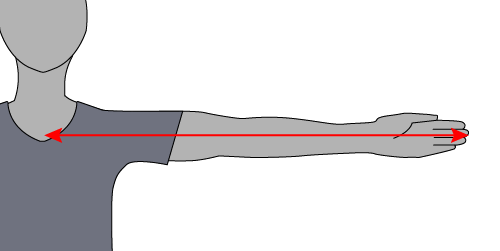1. Arm length
Measured from the rear side of the patient, it's the distance between the upper edge of the acromion process of the scapula and the bony part of the mid-elbow.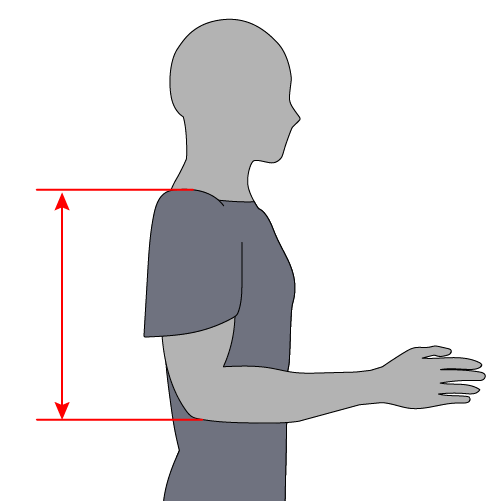1. Recumbent height (or crown-to-heel length)
It is the patient's height while they're recumbent; or the distance between the top of the head to the heels. It requires a special measuring scale.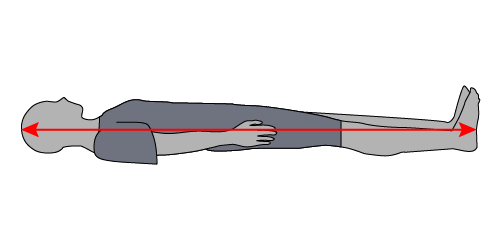1. Knee height
It is measured using a sliding caliper. The patient is lying supine with legs bare from just above the knee or sitting at the edge of a bed. One blade of a caliper is placed under the heel, and the other is placed on the anterior surface of the thigh.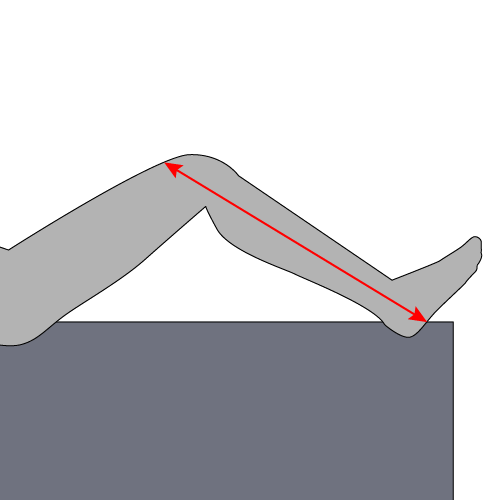1. Calf circumference
Take the measure in the non-dominant leg and aim for the widest circumference of the leg.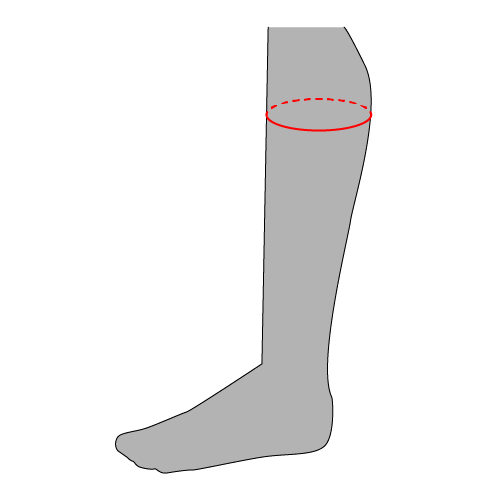1. Arm circumference
Measured in the mid-point of the arm, between the bony protrusion on the shoulder and the point of the elbow.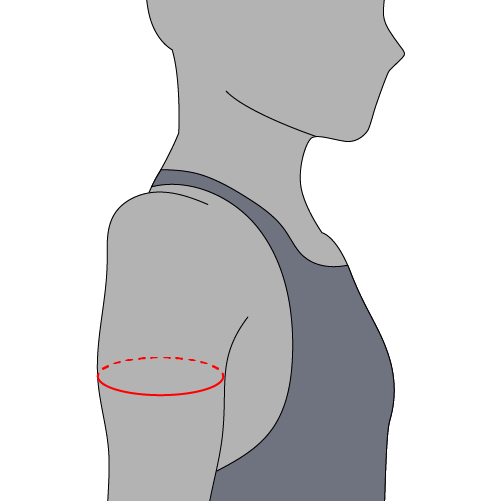1. Subscapular skinfold thickness
You need a caliper for that measure. It's the diagonal fold just below the inferior angle of the scapula.

Skinfold body fat calculator used to be a method to estimate a person's body fat. However, it's not seen as that valid anymore.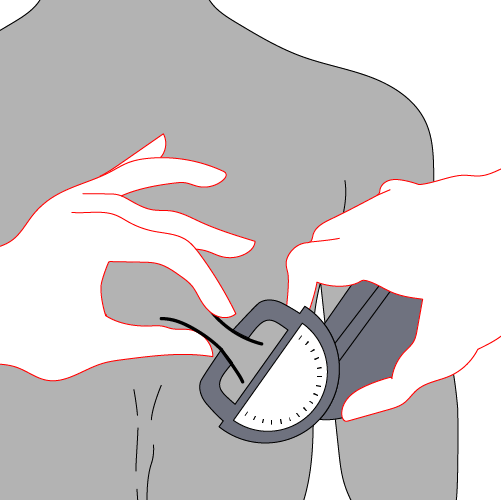1. Forearm length
The distance between the point of the elbow and the midpoint of the prominent wrist bone.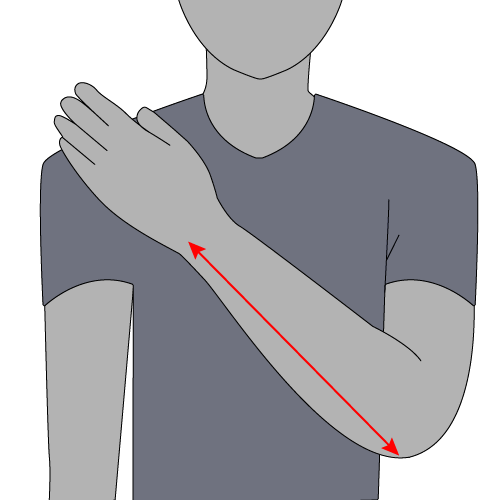1. Abdominal circumference
It's the circumference of your abdomen in the midpoint between the last rib and the bony part of your hip. The patient shouldn't be sucking in the stomach.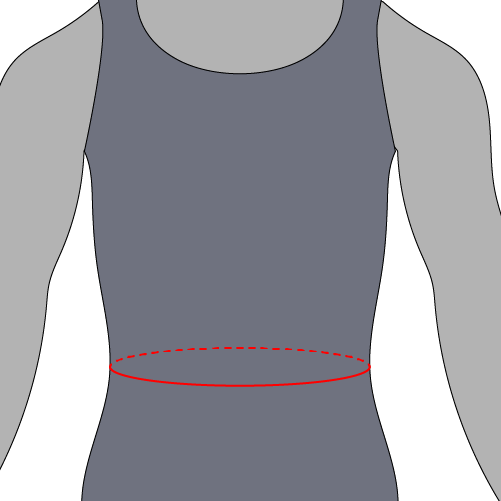💡 Abdominal, or waist circumference, is an important indicator of health and should not exceed 102 cm in men and 88 cm in women. Visit waist to hip ratio calculator to learn more.

## Estimating height formulas — how to estimate height without standing

Regarding alternatives to standing height measurements, luckily, we have quite a few options. See what formulas we're using to estimate the immobile patient's height:

1. Mitchell & Lipschitz formula

Height = Semi-span × 2

2. WHO formula (uses meters as a base unit)

Height = (0.73 × 2 × Half of arm span) + 0.43

3. Rabito et al. formula - two options

Height = 58.694 - (2.974 × Sex*) - (0.0736 × Age) + (0.4958 × Arm length) + (1.132 × Semi-span)

or

Height = 63.525 - (3.237 × Sex*) - (0.06904 × Age) + (1.293 × Semi-span)

* 1 — value for male; 2 — value for female.

4. Gray et al. formula

Height = Recumbent height

5. Chumlea et al. formula

White women
Height = 70.25 + (1.87 × Knee height) - (0.06 × Age)

Black women
Height = 68.1 + (1.86 × Knee height) - (0.06 × Age)

White men
Height = 71.85 + ( 1.88 × Knee height)

Black men
Height = 73.42 + (1.79 × Knee height)

6. Cereda et al. formula

Height = 60.76 + (2.16 × Knee height) – (0.06 × Age) + (2.76 × Sex**)

** 1 — value for male; 0 — value for female.

7. Knee height formula

Women
Height = 84.88 - (0.24 × Age) + (1.83 × Knee height)

Men
Height = 64.19 - (0.04 × Age) + (2.02 × Knee height)

8. Forearm formula

This is not a real equation but rather a chart. For every forearm (ulna) measurement of a person of certain sex and age, there is a corresponding estimated height. See more in the corresponding section.

9. Demi-span formula

Women
Height = (1.35 × Demi-span) + 60.1

Men
Height = (1.4 × Demi-span) + 57.8

If not marked differently, all the length measurements are in centimeters (cm), and the age is in years.

## Estimating weight formulas (without actually standing on a scale)

When a patient cannot stand, and you don't have a special scale to lay on, estimating weight formulas is the only way to figure out the person's weight. Here are the equations we use in height and weight measures in the bedridden patients calculator:

1. Chumlea et al. formula

Women
Weight = (1.27 × Calf circumference) + (0.87 × Knee height) + (0.98 × Arm circumference) + (0.4 × Subscapular skinfold thickness) - 62.35

Men
Weight = (0.98 × Calf circumference) + (1.16 × Knee height) + (1.73 × Arm circumference) + (0.37 × Subscapular skinfold thickness) - 81.69

2. Rabito et al. formula — three options

Weight = (0.503 × Arm circumference) + (0.5634 × Abdominal circumference) + (1.318 × Calf circumference) + (0.0339 × Subscapular skinfold thickness) - 43.156

Weight = (0.4808 × Arm circumference) + (0.5646 × Abdominal circumference) + (1.316 × Calf circumference) - 42.245

Weight = (0.5759 × Arm circumference) + (0.5263 × Abdominal circumference) + (1.2452 × Calf circumference) - (4.8689 × Sex*) ± 32.9241

* 1 — value for male; 2 — value for female.

3. Ross laboratories formula

White women
Weight = (1.01 × Knee height) + (2.81 × Arm circumference) - 66.04

Black women
Weight = (1.24 × Knee height) + (2.81 × Arm circumference) - 82.48

White men
Weight = (1.19 × Knee height) + (3.21 × Arm circumference) - 86.82

Black men
Weight = (1.09 × Knee height) + (3.14 × Arm circumference) - 83.72

If not marked differently, all the length measurements are in centimeters (cm), and the weight is given in kilograms (kg).

## Forearm length based height estimation charts

If you want to use the forearm (ulna) length to estimate the patient's height, use the table below. Measure the forearm length, and find the corresponding height depending on the sex and age of the patient.

 Height (cm) - men <65 yrs Height (cm) - men >65 yrs Ulna length (cm) Height (cm) - women <65 yrs Height (cm) - women >65 yrs 146 148 149 151 153 155 157 158 160 162 164 166 167 169 171 173 175 176 178 180 182 184 185 187 189 191 193 194 145 146 148 149 151 152 154 156 157 159 160 162 163 165 167 168 170 171 173 175 176 178 179 181 182 184 186 187 18.5 19 19.5 20 20.5 21 21.5 22 22.5 23 23.5 24 24.5 25 25.5 26 26.5 27 27.5 28 28.5 29 29.5 30 30.5 31 31.5 32 147 148 150 151 152 154 155 156 158 159 161 162 163 165 166 168 169 170 172 173 175 176 177 179 180 181 183 184 140 142 144 145 147 148 150 152 153 155 156 158 160 161 163 165 166 168 170 171 173 175 176 178 179 181 183 184

## FAQ

### How to estimate height without standing with the WHO formula?

To calculate height:

1. You need to provide the half-arm span length. Let's say it's equal to 91 cm.

2. WHO formula:

Height [m] = (0.73 × 2 × Half of arm span) + 0.43

3. Convert the half-arm span to meters:

91 cm = 0.91 m

4. Plug in the value:

Height = (0.73 × 2 × 0.91) + 0.43 = 1.76

5. Height is equal to 1.76 m. You can double-check with the bedridden patient height calculator.

### What are the formulas for estimating weight?

Formulas for estimating weight include:

• Chumlea et al. formula (you need: arm circumference, calf circumference, knee height, and subscapular fold thickness);
• Rabito et al. formula (measurements needed: arm circumference, calf circumference, and abdominal circumference); and
• Ross laboratories formula (you'll need the person's race, arm circumference, and knee height).

### How to calculate height if the recumbent height is 167 cm?

The patient's height is 167 cm.

1. For that calculation, you can use Gray formula:

Height = recumbent height

2. The actual height is equal to the recumbent height.

3. The units don't matter; you can use whichever is comfortable.

4. In our case:

Height = recumbent height = 167 cm

### What are alternatives to standing height measurements?

Alternatives to standing are either measuring recumbent height using a special scale (following the Gray formula) or estimating height with one of the formulas:

• Mitchell & Lipschitz formula (uses semi-span length);
• WHO formula (uses half arm span length);
• Rabito et al. formula (needed: arm length and semi-span);
• Chumlea et el. formula (uses knee height);
• Cereda et al. formula (knee height again);
• Knee height formula;
• Forearm (ulna) length formula; or
• Demi-span formula.

### How to measure the height and weight of an immobile patient?

The only way to measure the height and weight of an immobile patient without using specialized equipment is to measure indirectly. You can obtain certain measurements that don't require standing or forced positions, such as knee height, arm circumference, and many more. Check the formulas estimating height and weight and choose the most appropriate one.

Anna Szczepanek, PhD and Aleksandra Zając, MD
Height estimation
Study
Mitchell & Lipschitz
Semi-span
cm
Height
cm
Weight estimation
Study
Ross laboratories
Sex
Male
Race
White
Arm circumference
cm
Knee height
cm
Weight
kg
People also viewed…

### BMI in pregnancy

Everything you should know about pregnancy weight gain, pre-pregnancy BMI, and the risks connected to obesity in pregnant women.

### LDL cholesterol

The LDL calculator uses the Friedewald equation to find the blood level of low-density lipoproteins.

### Plant spacing

Planning out your garden? Try the plant spacing calculator.

### Secretary problem (Valentine's day)

Use the dating theory calculator to enhance your chances of picking the best lifetime partner.